## Access NCERT Solutions for Class 6 Maths Chapter 13: Symmetry Exercise 13.3

### Access NCERT Solutions for Class 6 Maths Chapter 13: Symmetry Exercise 13.3

1. Find the number of lines of symmetry in each of the following shapes. How will you check your answers?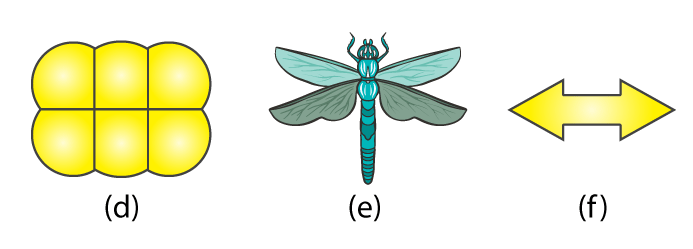Solutions:

(a) For the given figure there are 4 lines of symmetry.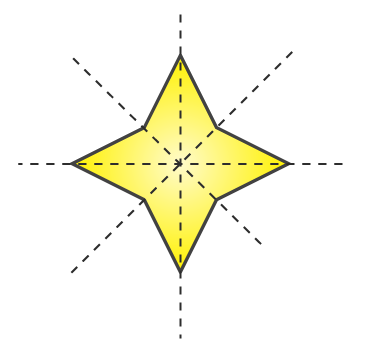(b) For the given figure there is only 1 line of symmetry.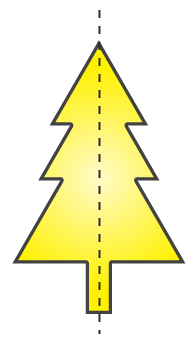(c) For the given figure there are 2 lines of symmetry.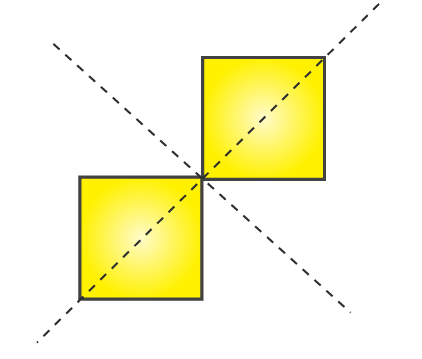(d) For the given figure there are 2 lines of symmetry.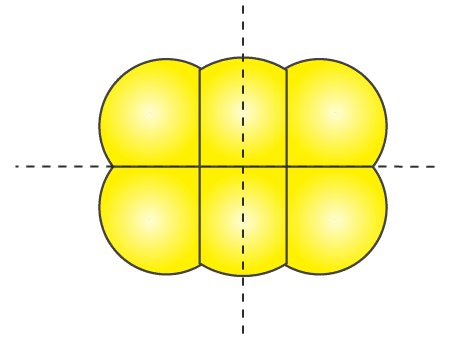(e) For the given figure there is only 1 line of symmetry.(f) For the given figure there are 2 lines of symmetry.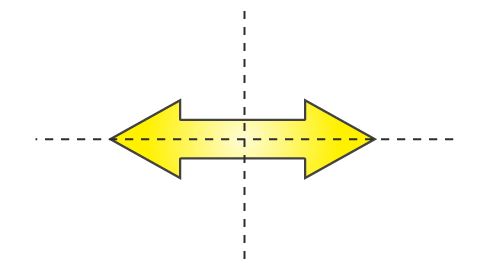2. Copy the following drawing on squared paper. Complete each one of them such that the resulting figure has two dotted lines as two lines of symmetry.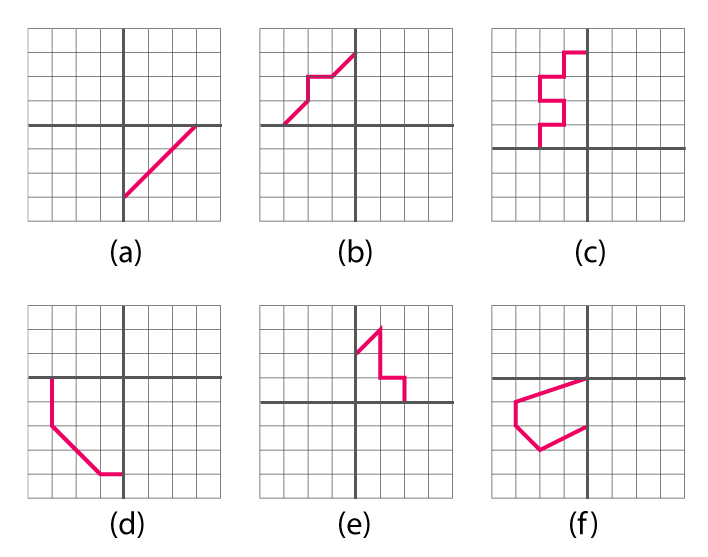How did you go about completing the picture?

Solutions:

We can complete these figures by drawing similar parts as shown in these figures. First about the vertical line of symmetry and then about the horizontal line of symmetry or first about the horizontal line of symmetry and then about the vertical line of symmetry.

Completed figures are as follows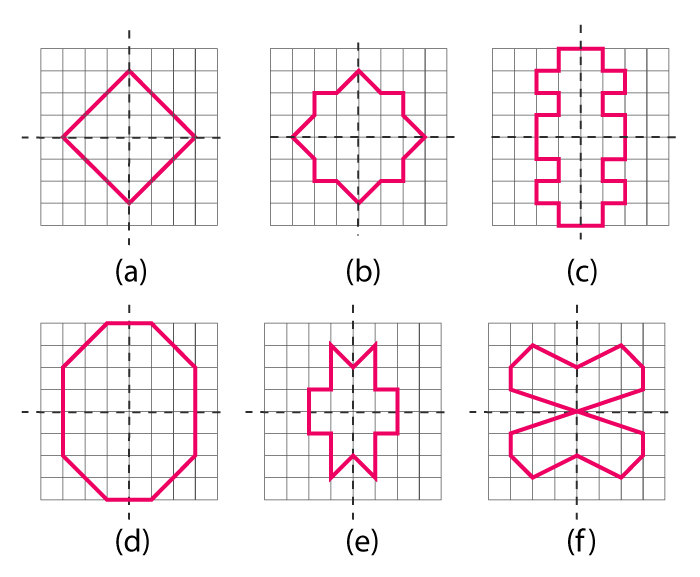3. In each figure alongside, a letter of the alphabet is shown along with a vertical line. Take the mirror image of the letter in the given line. Find which letters look the same after reflection (i.e. which letters look the same in the image) and which do not. Can you guess why?

Try for O E M N P H L T S V X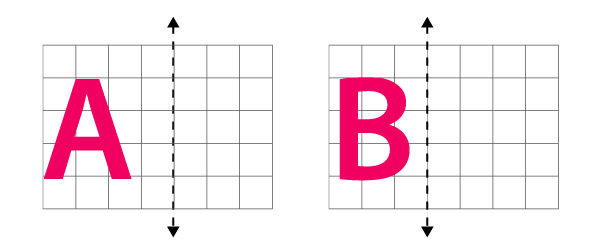Solutions:

The mirror images of these figures are as followsThe letters having vertical line of symmetry will have same mirror images. These letters are O, M, H, T, V, X and thus these letters will look the same.